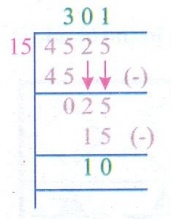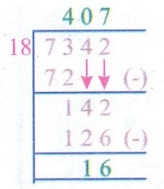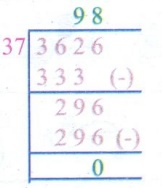Home | | Maths 5th Std | Exercise 2.9 (Divide 4 digits by 2 digits)

# Exercise 2.9 (Divide 4 digits by 2 digits)

Text Book Back Exercises Questions with Answers, Solution : 5th Maths : Term 1 Unit 2 : Numbers : Exercise 2.9 (Divide 4 digits by 2 digits)

Exercise 2.9

a. A cement factory produces 37500 bags of cements in a month (30 days). How many cement bags are produced in one day?

Solution:

Number of cement bags produced in 30 days = 37500

Number of cement bags produced in 1 day = 37500÷30Number of cement bags produced in one day = 1250

b. 8075 mangoes are harvested in a mango garden. 95 mangoes are packed in a bag. How many mango bags will be there?

Solution:

95 mangoes are packed in = 1 bag

8075 mangoes are packed in = 8075÷9585 Mango bags will be there

c. 25 families living in a street need 1625 liters of water per day. How much of water is needed for a family?

Solution:

Water needed for 25 families = 1625 L

Water needed for 1 family = 1625 ÷ 25Water needed for 1 family = 65 litres

d. 6750 bananas have to be loaded in a tempo van. If 15 bananas were arranged in one baskets, then how many baskets will be needed to arrange all the bananas?

Solution:

Bananas arranged in 15 baskests = 6750

Bananas arranged in one baskets = 6750 ÷ 15Bananas arranged in one basket = 450

II. Divide the following

a. 4525 ÷ 15

b. 3448÷24

c. 7342÷18

d. 3626÷37

e. 4872÷56

a. 4525 ÷ 15Quotient = 301

Remainder = 10

b. 3448÷24Quotient = 433

Remainder = 16

c. 7342÷18Quotient = 407

Remainder = 16

d. 3626÷37Quotient = 98

Remainder = 0

e. 4872÷56Quotient = 87

Remainder = 0

Tags : Numbers | Term 1 Chapter 2 | 5th Maths , 5th Maths : Term 1 Unit 2 : Numbers
Study Material, Lecturing Notes, Assignment, Reference, Wiki description explanation, brief detail
5th Maths : Term 1 Unit 2 : Numbers : Exercise 2.9 (Divide 4 digits by 2 digits) | Numbers | Term 1 Chapter 2 | 5th Maths